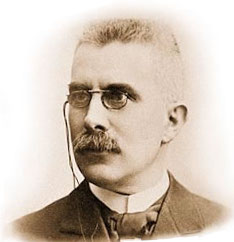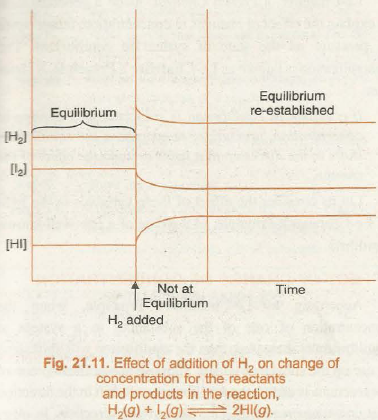# FACTORS AFFECTING THE POSITION OF EQUILIBRIUM IN ACCORDANCE WITH THE LE’ CHATELIER’S PRINCIPLE

## Le Chatelier’s Principle

Le Chatelier’s principle states that changes to an equilibrium system will result in a predictable shift that will counteract the change.Henry Le Chatelier: A photograph of Henry Le Chatelier.

# Factors Affecting Equilibria- Le-Chatelier’s Principle

Le-Chatelier, a French Chemist, made a generalization to explain the effect of changes in concentration, temperature or pressure on the state of system in equilibrium. The generalization is known as Le-Chatelier’s Principle. It states that:

If a system in equilibrium is subjected to a change of concentration, pressure or temperature, the equilibrium shifts in the direction that tends to undo the effect of the change.

Let us consider the effect of these variables in the light of Le-Chatelier’s Principle, in the case of a few well known equilibria.

EFFECT OF CHANGE OF CONCENTRATION

According to Le-Chatelier’s Principle, when the concentration of one of the substances in a system at equilibrium is increased, then the equilibrium will shift so as to use up the substance added. Suppose at equilibrium one of the reactants is added, the equilibrium will shift in the direction that consumes reactants, i.e., the forward direction. In other words, more of the reactants would be converted into products. On the other hand, if one of the products is added, the equilibrium will shift in the backward direction because it consumes the products.

In order to understand the effect of change of concentration on equilibrium, let us consider the reaction,If H2 is added to the reaction mixture at equilibrium, then The equilibrium of the reaction is disturbed. In order to restore it, the reaction proceeds in a directions wherein H2 is consumed, i.e., more of H2 and 12 react to form HI and finally the equilibrium is re-established. Thus, addition of H2 shifts the equilibrium in forward direction. In the new equilibrium mixture, the concentration of H2would be less than it was after addition of H2 but more than what it was in the original equilibrium (Fig. 21.11).

The same point can be understood in terms of the reaction quotient, Qc.

Qc = [HI]2 / [H2] [I2]

If concentration of H2 is increased, the value of Qc decreases and it is no longer equal to Kc. In order to attain . new equilibrium, the reaction proceeds in forward direction resulting in decrease in concentrations of H2 and 12 and increase in concentration of HI. Finally, new equilibrium is attained.Similarly, we can say that removal of a product also favours the forward reaction and increases the concentration of the products. For example, in the large scale production of CaO, by calcination of lime stone (CaCO3), CO2 is continuously removed from the klin to shift the reaction in forward direction. Continuous removal of one of the products maintains Qc at a value less than Kc and thus reaction keeps on proceeding in the forward direction.

The effect of concentration on equilibrium can be demonstrated with the help of the following reaction:A reddish colour appears on adding 2-3 drops of0.002 M potassium thiocynate solution to 5 mL of 0.2 M iron(ID) trioxonitrato (V) solution due to the formation of [Fe(SCN)]2+. The intensity of the red colour becomes constant when equilibrium is attained. This equilibrium can be shifted in either forward or backward directions depending on our choice of adding a reactant or a product.

Suppose, some iron(ill) salt is added to this equilibrium. The colour of the solution deepens immediately showing that there is increase in the concentration of the coloured complex ion [FeSCN]2+. This change is in accordance with Le-Chatelier’s principle. Addition of more of Fe3+ ions has resulted in increasing the concentration of the complex [FeSCN]2+ ions. Similarly, if some potassium thiocyanate is added at the equilibrium, the colour deepens again due to the formation of more of the [FeSCN]2+ ions.

The above equilibrium can be shifted in the backward direction by adding reagents that remove Fe3+ or SCN- ions. For example, oxalic acid (H2C20 4), reacts with Fe3+ ions to form the stable complex ion [Fe(C2OH3]3-, thus decreasing the concentration of Fe3+ions. In accordance with the Le-Chatelier’s principle, the decrease in the concentration of Fe3+ ions, leads to the decrease in concentration of [FeSCN]2+ ions which is indicated by the fact that colour of the solution becomes lighter.

EFFECT OF CHANGE IN PRESSURE

The change of pressure has effect only on those equilibria which involve gaseous substances and proceed with a change in the number of moles of the gases. According to Le-Chatelier’s principle, increase of external pressure should affect the equilibrium in such a way as to reduce the pressure. This implies that the equilibrium will shift in the direction which has smaller number of moles of the gaseous substances. This can be easily understood from the following equilibrium representing the formation of ammonia.On increasing the pressure, the volume occupied by the system will decrease. As such there will be greater number of moles per unit volume. The effect of this change can be counteracted if the equilibrium shifts in the direction involving a decrease in the number of moles. This can happen only if nitrogen and hydrogen combine to form ammonia. Thus, increase in pressure in this case will favour the forward reaction

This can also be understood in terms of reaction quotient Qc. Suppose pressure of the reaction mixture is doubled and volume is reduced to half. The partial pressures and concentrations of various species in the reaction mixture would become double. We obtain Qc by replacing each equilibrium concentration by double its value.

KC = [NH3]2 / [N2] [H2]3 = (a)2 / (b) (c) = a2 / bc3

Q= [NH3]2 / [N2] [H2]3 =  (2a)/ (2b) (2c)3 = a2 / 4bc3

As the value of~ is less than Kc, the  reaction proceeds in the forward direction.

Now, let us consider some reactions which do not involve any change in number of moles of gaseous species:In such reactions pressure does not have any effect on equilibrium.

Let us explain the effect of pressure on solubility of gases in liquid solvents on the basis of Le-Chatelier’s principle. As an example, let us study the equilibrium involving dissolution of CO2 in water. The equilibrium may be represented as:On increasing the pressure of CO2, the equilibrium shifts in the direction which results in the decrease in pressure of CO2. The pressure of CO2 will be lowered only if CO2(g) dissolves in water to form CO2(aq). Thus, solubility of a gas in some liquid is directly proportional to the pressure of the gas in equilibrium with the solution.

EFFECT OF TEMPERATURE

A chemical equilibrium involves two opposing reactions, one favouring the products and the other favouring the reactants. If one of the reactions is exothermic the other must be endothermic. For example, let us consider the equilibrium representing the formation of ammonia:In this equilibrium, the forward reaction is exothermic while the backward reaction is endothermic. Now, if the temperature is increased, the equilibrium will shift in the direction of endothermic reaction, which tends to undo the effect of added heat. Since the backward reaction is endothermic, so the equilibrium shifts in favour of backward reaction. In other words, it will result into lesser amount of ammonia. On the other hand, if temperature is decreased, the equilibrium will shift towards exothermic reaction. So low temperature favours the formation of ammonia.

EFFECT OF CATALYST

The presence of catalyst does not disturb the state of equilibrium because it increases the rate of forward as well as backward reaction to the same extent. It simply hastens the attainment of equilibrium by providing a new pathway having low activation energy. Catalyst does not disturb the equilibrium composition of the reaction mixture.

Certain reactions such as formation of ammonia from nitrogen and hydrogen cannot be carried out at high temperatures because high temperature favours the backward reaction. So such reactions give poor yields at high temperatures and therefore, have to be carried out at low temperature. But at low temperature the rate of reaction becomes very slow and it takes very long time to attain the equilibrium. In order to increase the rates of such reactions, generally, catalysts are used so that equilibrium is attained early. For example, in case of formation of ammonia, a catalyst consisting of iron, is used to catalyse the reaction.

## STEM Elearning

We at FAWE have built this platform to aid learners, trainers and mentors get practical help with content, an interactive platform and tools to power their teaching and learning of STEM subjects, more

#### How to find your voice as a woman in Africa

© FAWE, Powered by: Yaaka DN.